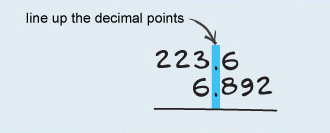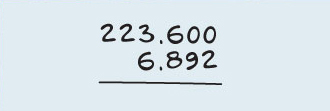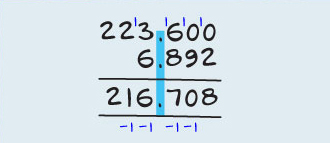Numbers: An introduction to subtraction

This free course is available to start right now. Review the full course description and key learning outcomes and create an account and enrol if you want a free statement of participation.

Free course

# 7 Subtracting decimals by lining them up

Subtracting whole numbers such as 52 from 375 is fairly straightforward. Subtracting decimal numbers such as 6.892 from 223.6 uses the same process but with one extra step – you have to line the decimal points up first.

Rather than arranging your two numbers so that they line up on the right-hand side, you need to line up the decimal points, regardless of how many numbers there are after the decimal point. In the example below, the top number has one number after the decimal point. It is said to have one decimal place. But the bottom number has three decimal places.In any columns that are ‘missing’ numbers after the decimal point, assume that the missing number is zero, like this.Now do the subtraction, starting on the right as usual, and borrowing from the next column where necessary.

When you have finished the calculation, remember to put the decimal point into your answer below the line, in the same position as the two numbers you are subtracting.

So the above subtraction when it is completed, with all its borrowings shown and with all its decimal points lined up, looks like this.Sub_1

### Take your learning further

Making the decision to study can be a big step, which is why you'll want a trusted University. The Open University has 50 years’ experience delivering flexible learning and 170,000 students are studying with us right now. Take a look at all Open University courses.

If you are new to university level study, find out more about the types of qualifications we offer, including our entry level Access courses and Certificates.

Not ready for University study then browse over 900 free courses on OpenLearn and sign up to our newsletter to hear about new free courses as they are released.

Every year, thousands of students decide to study with The Open University. With over 120 qualifications, we’ve got the right course for you.

Request an Open University prospectus